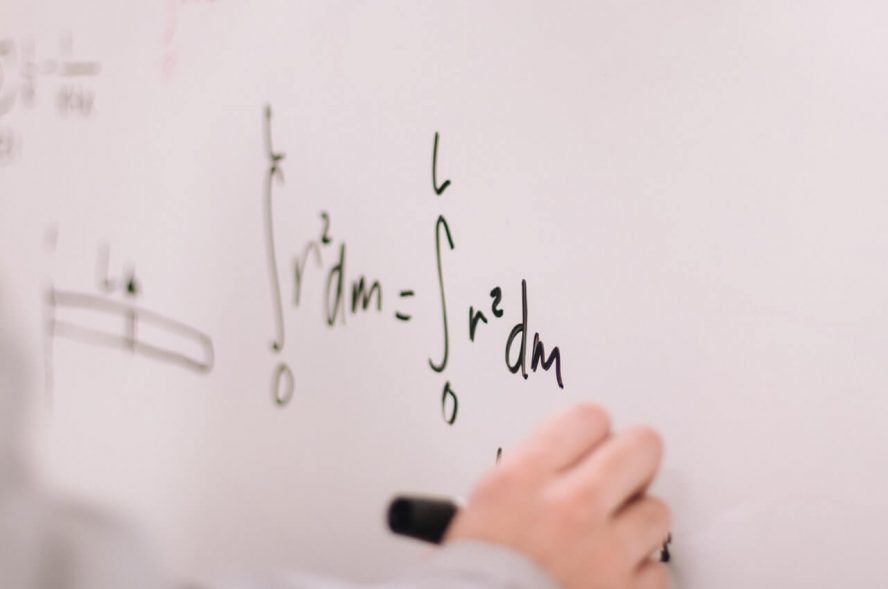# Top 5 Hardest GCSE Maths Questions in 2022

In GCSE by Think Student Editor20 Comments

Today we are going to be looking over at some of the toughest GCSE Maths questions out there. We have tried our best to ask GCSE students what they thought was the hardest maths question that they have come across during their time at school. However, in reality, everyone has their own unique strengths and weaknesses and therefore some will agree with this list and others will not – there is no such thing as “the hardest GCSE Maths question”, due to the fact that different questions suit different student’s unique skillsets.

Due to the aforementioned reason, make sure that you take this list with a pinch of salt as it will undoubtedly not apply to all students. Lastly, if you have come across a question that you think deserves to be on this list, let us know by filling in the form below. Without further ado, let’s get into the hardest GCSE Maths questions out there!

## 5. Edexcel June 2018 Higher Paper 1 – Question 6

This question takes position number five on our list of the hardest GCSE Maths questions. It may look easy on the first glance, however it has made many students want to curl and run away in the exam… You can see a picture of this question, taken from the official Edexcel June 2019 Higher Paper 1, below.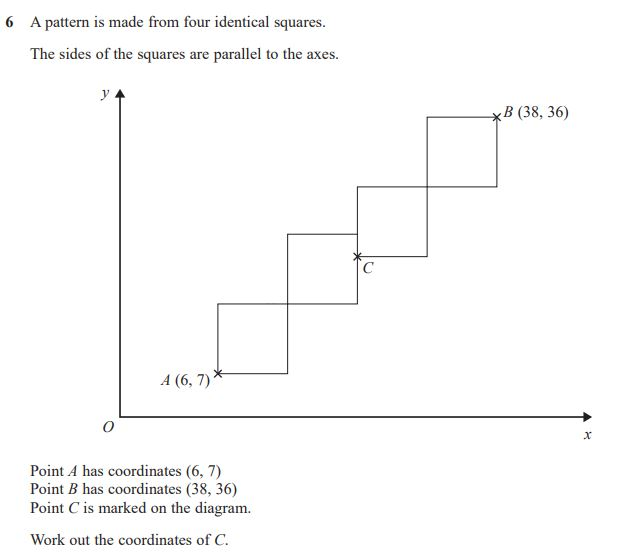This question actually doesn’t rely on any complicated mathematics and only elementary knowledge of coordinate geometry is required. The question displays a graph containing multiple points that form four identical squares. You are given the coordinates of two points, A and B, that lie on the corners of the furthest away squares and you need to find an intermediary point labelled C (see diagram).

It’s worth noting that the exam board is never trying to trick you and that they do want to give students a fair chance at displaying their knowledge in the exam. That said, many questions often contain an element that you need to look for, that is not immediately obvious. In this question, the “trick” or element that you need to recognise is that the point labelled C has the following properties:

• It does not lie on the midpoint of either square it is adjacent to.
• It represents the point at which the squares no longer are connected via just their corners.

The mark scheme section that relates to this particular question can be seen below.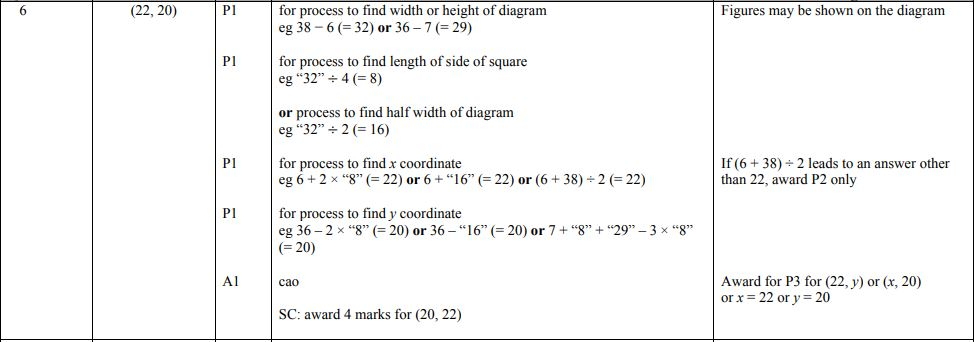As you can see, the solution is fairly simplistic and nothing a competent GCSE Maths student would struggle with in the classroom. That said, in the heat of the exam, anyone can be stressed enough to miss finding the pattern and relationships within this question and that’s what makes this question one of the hardest.

To download the paper that contains this particular question, go to the Edexcel past paper page, which you can find here.

## 4. AQA June 2017 Higher Paper 1 – Question 29

To those GCSE Maths students who see themselves as trigonometry specialists, this question may not be that hard in your opinion. That said, to those students who tend to struggle with trigonometry, this question is definitely challenging. An image of this question can be found below.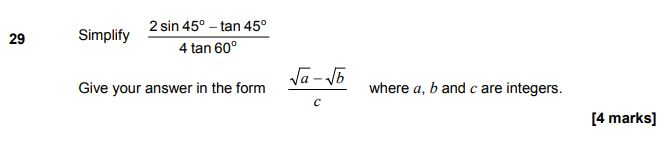So, there’s two parts of this question. The first is to convert the sin and tan terms into a format that will enable you to simplify the equation. The second is to rearrange the equation until you get to the format specified within the question. This question tests two different mathematical skillsets and therefore, if you aren’t familiar with the trigonometry section of the GCSE Maths specification, it may very well catch you out.

When tackling this question, you need to make sure that you have remembered all your trigonometric values, as a calculator is not allowed for this question. This means that you will be expected to convert the trigonometric terms into values all by yourself, without external support.

Once you have substituted in your calculated values to replace the scary trigonometry, you mustn’t forget that you need to convert the equation into a specific form! For this, you will need to know how to rationalise denominators as the substituted equation will leave you with a surd within the denominator.

To see how both of these skills are implemented to solve this question, the mark scheme section that relates to this particular question can be seen below.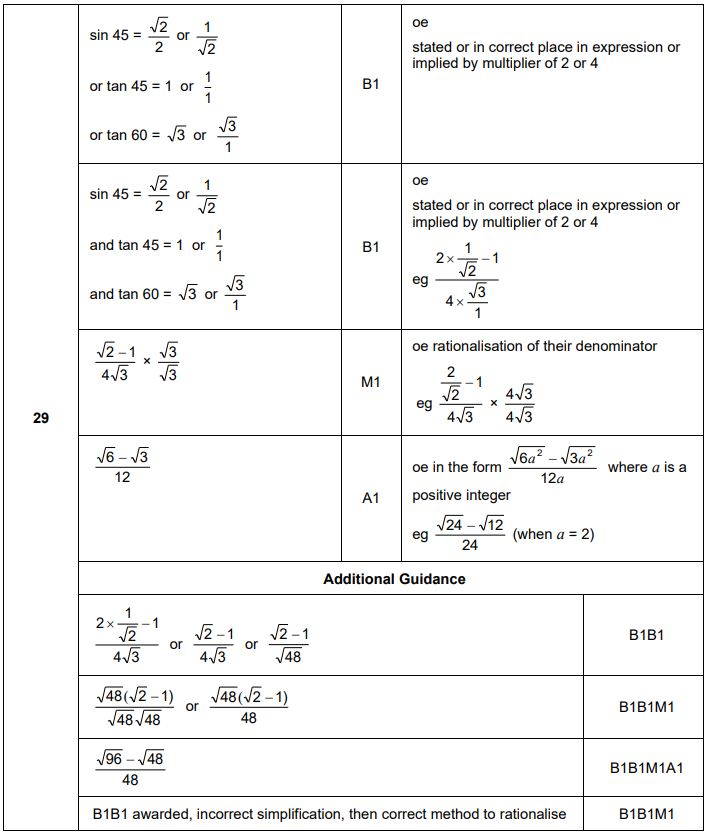The AQA mark scheme for this question lays out the solution fairly clearly in my opinion, so I won’t ramble on too much about how it was solved. However, I have listed some super helpful revision resources that you should check out before tackling this question at the bottom of this article.

To download the paper that contains this particular question, go to the AQA GCSE Maths past paper page, which you can find here.

## 3. AQA June 2017 Higher Paper 3 – Question 13

This questions feels relatively simple and the amount space the exam board have given you to work it out certainly suggests that it should be simple, however, when we were asking students what GCSE Maths question they found the hardest, this one came up multiple times. Due to this, it has of course made it onto the list.

In my opinion, it is not this question specifically that students find difficult, but in fact this type of question. Determining overall mean averages from the mean averages of sub-groups is something students really do struggle with. An image of this question is shown below.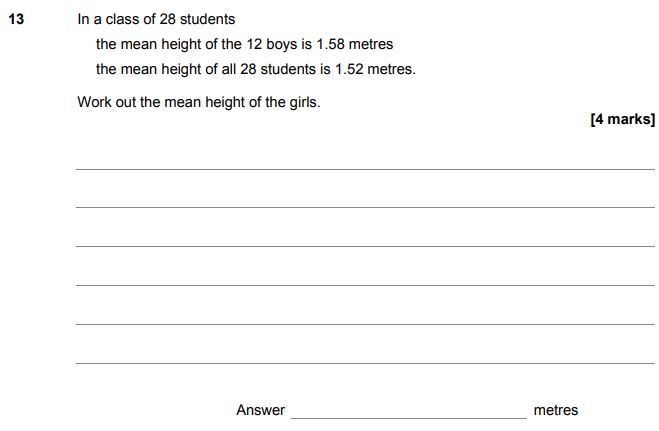In this question, we are given the information that there is a class with an overall population of 28. Out of the 28 students, 12 are boys and therefore, we can deduct that 16 are girls. Out of the 12 boys in the class, it is stated that the mean average height is 1.58m. Alongside this information, we are told that the mean average height of both the girls and the boys combined is 1.52m. This question asks us, from the information given, to deduce the mean average height of just the girls.

So, what’s so difficult about this? Well, in reality if you have revised an effective method for solving these types of questions, nothing is difficult about this question. Therefore, if you haven’t already done so, study these types of questions right now, before you take your GCSE Maths exams!

There are two different methods that you can use to solve this question:

1. Use the calculated number of girls in the class within your calculations and set the sum of the heights of the girls within the class to 16x. 16 being the amount of girls in the class and x representing the mean average height, that the question asks us to solve.
2. Use only the direct numbers stated within the question calculate the sum of the heights of the girls within the class. This method involves calculating the sum of the heights of the boys and the sum of the heights of the entire class and finding the difference between them. This difference will be equal to the sum of the heights of the girls in the class.

After using either of the methods stated above you can follow out the routine shown in the mark scheme for this question below to get your solution.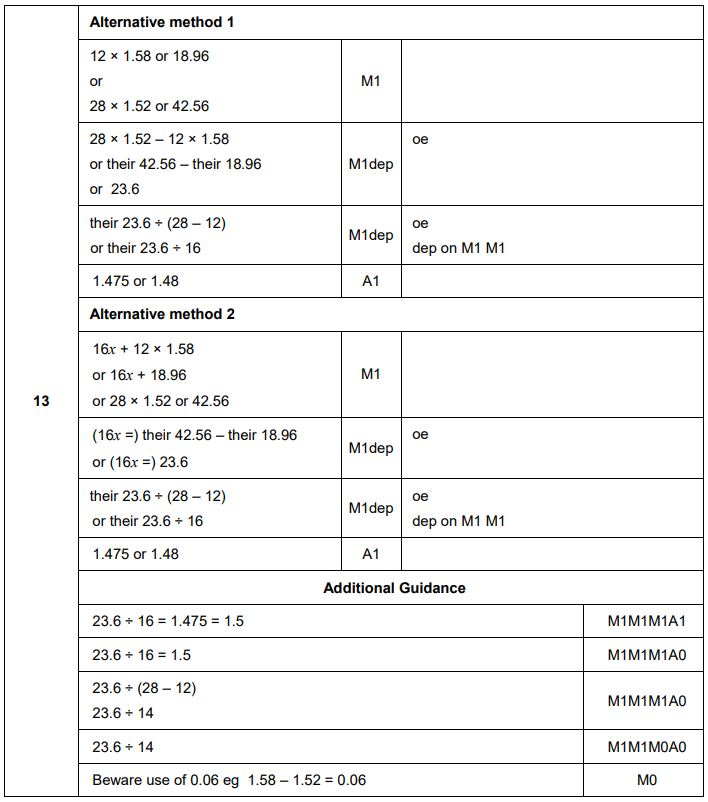As seen above, with the correct method, this question does not deserve the reputation it gets within the student world!

To download the paper that contains this particular question, go to the AQA GCSE Maths past paper page, which you can find here.

## 2. Edexcel June 2017 Foundation Paper 3 – Question 19

You read that right, this next question is on a foundation tier GCSE Maths paper. That said, it is my understanding that it was also included within the higher paper. Nevertheless, this question is abstract in its design and appears to be very simple from the illustrative diagram that the exam board provides, but is this first glance instinct correct? The question is shown below.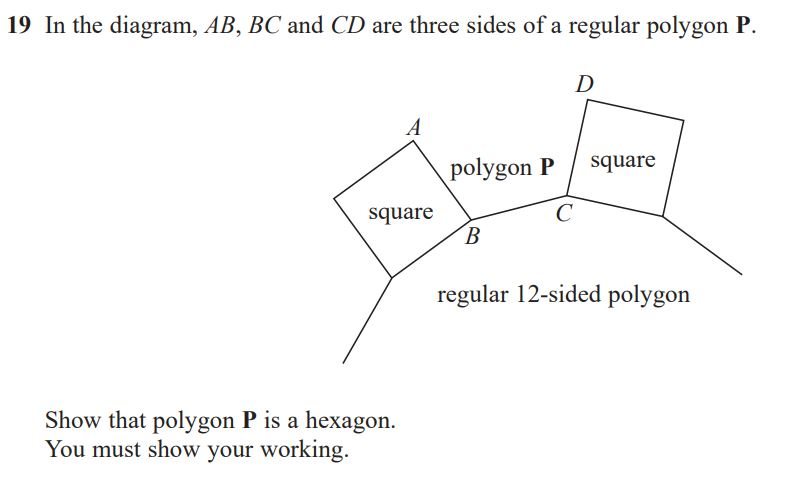So, there it is. In my opinion, this question looks fairly different from many other GCSE Maths questions and due to this I am classing this question as an abstract one. Much like some of the other questions on this list, I don’t actually feel that the mathematical skills that are required to solve this question are of that high standard. Instead, the question falls into the problem solving category, whereby, if you can spot the pattern and see how the shapes interact with each other, it all falls into place very easily.

Three uniquely different shapes are seen in this question and they are listed below:

• Square
• Regular 12-sided polygon
• Polygon with a currently unknown quantity of sides

The objective of this question is to, from the diagram provided, deduce the amount of sides’ polygon P has and therefore, prove it is a hexagon.

When you first see this question, your mind might go blank immediately. However, after gazing for a few minutes the thought of interior angles should pop into your head. Interior and exterior angles are fundamentally what this question is all about. If you are wanting to take a look at how this question is solved, the mark scheme for this specific question is shown below.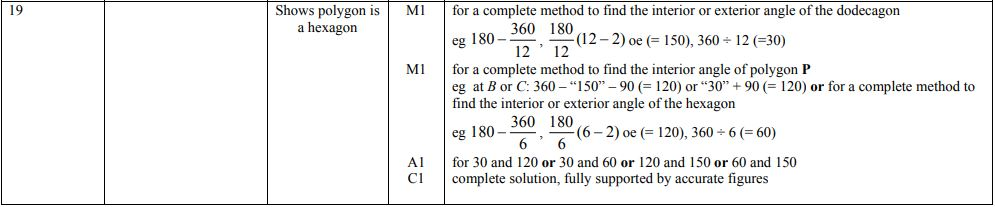The scary part of this question and mark scheme for most students was calculating the interior and exterior angles of a dodecagon (aka a polygon with twelve sides). If you equip yourself with a handy formula for calculating these types of angles for different types of polygons, you will find questions like this significantly easier to solve. To learn about these types of formula, check out our list of helpful resources at the bottom of this article.

To download the paper that contains this particular question, go to the Edexcel past paper page, which you can find here.

## 1. Edexcel June 2017 Higher Paper 1 – Question 22

This question looks tough, is tough and there actually isn’t anything you can do to make it easy quickly. The only thing you can do to tackle this question effectively in the exam is to practise GCSE Maths as much as humanly possible on the lead up to your exam. After all, you can never prepare for a single question as you don’t know what is going to come up, therefore, it is always best practise to assume the worse and leave no mathematical topic untouched when it comes to revision. The hardest question currently ranked on this list is shown below.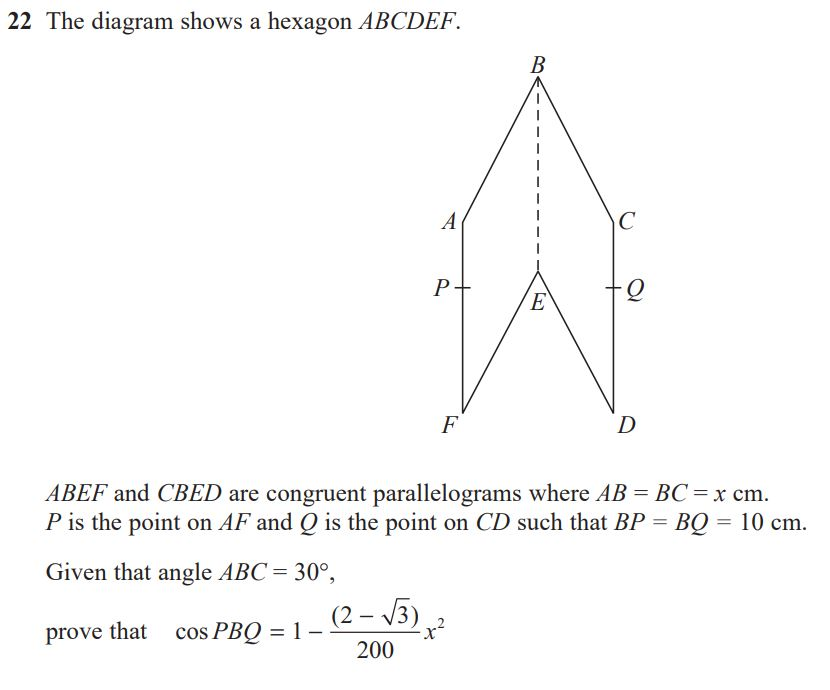It definitely looks scary and it requires knowledge from multiple different parts of the GCSE Maths specification to solve. For this question, a knowledge of the properties of parallelograms is most certainly going to come in handy. In addition to this, you must know how manipulate the cosine rule and use it effectively.

The shapes involved in this question are two identical parallelograms that when adjacent in the way identified in the diagram form a stretched deltoid-like shape. Within the question’s brief, you are told that the parallelograms are congruent, which confirms what we previously said about the shapes and we are also told that the lengths AB and AC are equal to the unknown value represented by the letter x. Lastly, we are told that the angle at B, enclosed by sides AB and BC, is 30 degrees and that the lengths BP and BQ are equal to 10.

With all that information flying around, it is going to be a really tough question for students to solve in the heat of the exam. In addition to that, it is the last question on this particular paper meaning that students will also be fatigued from solving everything else before.

To download the paper that contains this particular question, go to the Edexcel past paper page, which you can find here.

Without further ado, below is the mark scheme for this particular question.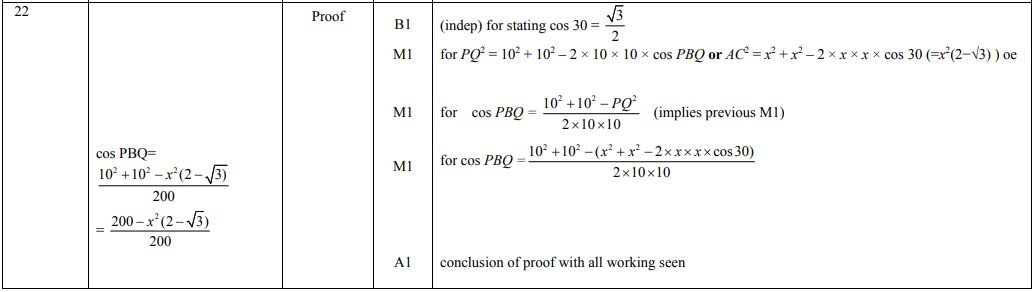So there it is, what did you think of these questions? If you disagree, drop a comment below letting us know what GCSE Maths questions you think are the hardest!

## Helpful Resources to Tackle the Hardest GCSE Maths Questions

 Skill Learning Resources Congruency Revision Maths Interior and Exterior Angles Dummies Cosine Rule BBC Bitesize Trigonometric Values BYJU’s BBC Bitesize Rationalising Denominators BBC Bitesize Math is Fun Corbett Maths
Article Rating
Subscribe
Notify ofInline Feedbacksflynn
1 year ago

helloThanish
1 year ago

wagwan7 months ago

Hi5 months ago

no10 months ago

not hard8 months ago

snjohn fortnite
8 months ago

facts ive seen questions 10 times harder

Last edited 8 months ago by john fortnite1 month ago

nig7 months ago

Yo

Last edited 7 months ago by Peter Filegulcin
6 months ago

lolballs
6 months ago

I had a sufficient method to work out these questions4 months ago

suiiiiiiiiiiiiiiiiiiiiiiiiiiiAhmaa
2 months ago

SUIIIIIIIIIIIIIIIIIIIIIIIIIIIIIIIIIIIIIIIIIIIIIIIIIIIIIthe beast
3 months ago

eazy gg bosjude
2 months ago

i got first one right and i am 112 months ago

ok king i did my alevel maths at 111 month agoname
1 month ago

these questions are so ezben dover
1 month ago

ez